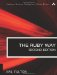# Section 9.2. Working with Stacks and Queues

### 9.1. Working with Sets

We've already seen how certain methods of the Array class let it serve as an acceptable representation of a mathematical set. But for a little more rigor and a little tighter coding, Ruby has a Set class that hides more of the detail from the programmer.

A simple require makes the Set class available:

`require 'set'`

This also adds a to_set method to Enumerable so that any enumerable object can be converted to a set.

Creating a new set is easy. The [] method works much as for hashes. The new method takes an optional enumerable object and an optional block. If the block is specified, it is used as a kind of "preprocessor" for the list (like a map operation).

`s1 = Set[3,4,5]                  # {3,4,5} in math notation arr = [3,4,5] s2 = Set.new(arr)                # same s3 = Set.new(arr) {|x| x.to_s }  # set of strings, not numbers`

#### 9.1.1. Simple Set Operations

Union is accomplished by the union method (aliases are | and +):

`x = Set[1,2,3] y = Set[3,4,5] a = x.union(y)    # Set[1,2,3,4,5] b = x | y         # same c = x + y         # same`

Intersection is done by intersection or &, which is an alias:

`x = Set[1,2,3] y = Set[3,4,5] a = x.intersection(y)    # Set b = x & y                # same`

The unary minus is set difference, as we saw in the array discussion (section 8.1.9, "Using Arrays As Mathematical Sets").

`diff = Set[1,2,3] - Set[3,4,5]    # Set[1,2]`

Membership is tested with member? or include? as with arrays. Remember the operands are "backwards" from mathematics.

`Set[1,2,3].include?(2)    # true Set[1,2,3].include?(4)    # false Set[1,2,3].member?(4)     # false`

We can test for the null or empty set with empty? as we would an array. The clear method will empty a set regardless of its current contents.

`s = Set[1,2,3,4,5,6] s.empty?              # false s.clear s.empty?              # true`

We can test the relationship of two sets: Is the receiver a subset of the other set? Is it a proper subset? Is it a superset?

`x = Set[3,4,5] y = Set[3,4] x.subset?(y)         # false y.subset?(x)         # true y.proper_subset?(x)  # true x.subset?(x)         # true x.proper_subset?(x)  # false x.superset?(y)       # true`

The add method (alias <<) adds a single item to a set, normally returning its own value; add? returns nil if the item was already there. The merge method is useful for adding several items at once. All these potentially modify the receiver, of course. The replace method acts as it does for a string or array.

Finally, two sets can be tested for equality in an intuitive way:

`Set[3,4,5] == Set[5,4,3]    # true`

#### 9.1.2. More Advanced Set Operations

It's possible of course to iterate over a set, but (as with hashes) do not expect a sensible ordering because sets are inherently unordered, and Ruby does not guarantee a sequence. (You may even get consistent, unsurprising results at times, but it is unwise to depend on that fact.)

`s = Set[1,2,3,4,5] s.each {|x| puts x; break }    # Output: 5`

The classify is like a multiway partition method; it was the inspiration for our classify method in section 8.3.3, "The partition Method."

`files = Set.new(Dir["*"]) hash = files.classify do |f|   if File.size(f) <= 10_000     :small   elsif File.size(f) <= 10_000_000     :medium   else     :large   end end big_files = hash[:large]   # big_files is a Set`

The divide method is similar, but it calls the block to determine "commonality" of items, and it results in a set of sets.

If the arity of the block is 1, it will perform calls of the form block.call(a) == block.call(b) to determine whether a and b belong together in a subset. If the arity is 2, it will perform calls of the form block.call(a,b) to determine whether these two items belong together.

For example, the following block (with arity 1) divides the set into two sets, one containing the even numbers and one containing the odd ones:

`require 'set' numbers = Set[1,2,3,4,5,6,7,8,9,0] set = numbers.divide{|i| i % 2} p set #  #<Set: {#<Set: {5, 1, 7, 3, 9}>,  #<Set: {0, 6, 2, 8, 4}>}>`

Here's another contrived example. Twin primes are prime numbers that differ by 2 (such as 11 and 13); singleton primes are the others (such as 23). The following example separates these two groups, putting pairs of twin primes in the same set with each other. This example uses a block with arity 2:

`primes = Set[2, 3, 5, 7, 11, 13, 17, 19, 23, 29, 31] set = primes.divide{|i,j| (i-j).abs == 2} # set is: #<Set: {#<Set: {23}>, #<Set: {11, 13}>, #         #<Set: {17, 19}>,     #<Set: {5, 7, 3}>, #         #<Set: {2}>, #<Set: {29, 31}>}> # More compactly: {{23},{11,13},{17,19},{5,7,3},{2},{29,31}}`

This method is difficult and confusing, in my opinion. I recommend the use of classify instead, which I find simple and intuitive.

It's important to realize that the Set class doesn't always insist that a parameter or operand has to be another set. (Refer to the discussion of duck typing in Chapter 1 if this confuses you.) In fact, most of these methods will take any enumerable object as an operand. Consider this a feature.

Other incidental methods can be applied to sets (including all methods in Enumerable). I don't choose to cover things here such as flatten; for more information, consult http://ruby-doc.org/ or any other reference.The Ruby Way, Second Edition: Solutions and Techniques in Ruby Programming (2nd Edition)
ISBN: 0672328844
EAN: 2147483647
Year: 2004
Pages: 269
Authors: Hal Fulton

Similar book on Amazon

flylib.com © 2008-2017.
If you may any questions please contact us: flylib@qtcs.net